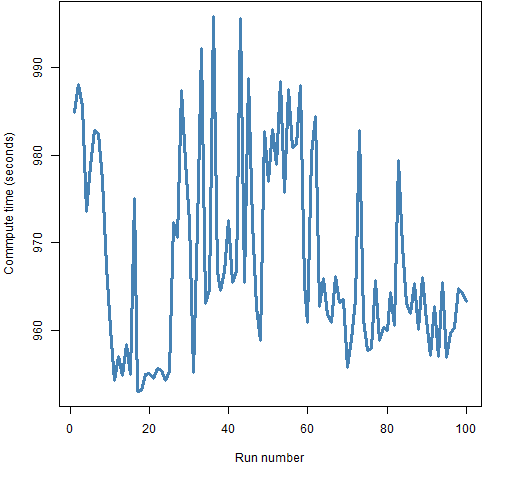# Posts Tagged ‘ simulated annealing ’

## Another comparison of heuristic optimizers

August 20, 2012
ByA herd of heuristic algorithms is compared using a portfolio optimization. Previously “A comparison of some heuristic optimization methods” used two simple and tiny portfolio optimization problems to compare a number of optimization functions in the R language. This post expands upon that by using a portfolio optimization problem that is of a realistic size … Continue reading →

## A comparison of some heuristic optimization methods

July 23, 2012
ByA simple portfolio optimization problem is used to look at several R functions that use randomness in various ways to do optimization. Orientation Some optimization problems are really hard. In these cases sometimes the best approach is to use randomness to get an approximate answer. Once you decide to go down this route, you need … Continue reading →

## [not] Le Monde puzzle (solution)

April 13, 2012
ByFollowing the question on dinner table permutations on StackExchange (mathematics) and the reply that the right number was six, provided by hardmath, I was looking for a constructive solution how to build the resolvable 2-(20,5,1) covering. A few hours later. hardmath again came up with an answer, found in the paper Equitable Resolvable Coverings by van

## simulated annealing for Sudokus 

March 16, 2012
ByOn Tuesday, Eric Chi and Kenneth Lange arXived a paper on a comparison of numerical techniques for solving sudokus. (The very Kenneth Lange who wrote this fantastic book on numerical analysis.) One of these techniques is the simulated annealing approach I had played with a long while ago.  They seem to use the same penalisation

## Harmonic means, again again

January 9, 2012
ByAnother arXiv posting I had had no time to comment is Nial Friel’s and Jason Wyse’s “Estimating the model evidence: a review“. This is a review in the spirit of two of our papers, “Importance sampling methods for Bayesian discrimination between embedded models” with Jean-Michel Marin (published in Jim Berger Feitschrift, Frontiers of Statistical Decision

## Le Monde puzzle 

January 10, 2011
ByFollowing the presentation of the first Le Monde puzzle of the year, I tried a simulated annealing solution on an early morning in my hotel room. Here is the R code, which is unfortunately too rudimentary and too slow to be able to tackle n=1000. #minimise \sum_{i=1}^I x_i #for 1\le x_i\le 2n+1, 1\e i\le I

## Le Monde puzzle [52|solution]

January 1, 2011
By$Le Monde puzzle [52|solution]$

I have now received the first issue of Le Monde magazine, including the solution to puzzle #52 I solved just in time by simulated annealing! The trick is in using the following theorem: Iter(1,x,y) is divisible by 10x-1 if and only if y is divisible by 10x-1. Then the value to be found is divisible

## Le Monde puzzle 

December 31, 2010
ByThe last puzzle of the year in Le Monde reads as follows (as far as I understand its wording!): Iter(n,x,y) is the function Iter=function(n,x,y){ if (n==1){ output=trunc(y/10)+x*(y%%10) }else{ output=Iter(n-1,x,Iter(1,x,y))} return output } Find the seven-digit number z such that Iter(6,1,z)=12, Iter(6,2,z)=19, Iter(6,3,z)=29, and Iter(6,-1,z)=Iter(6,-2,z)=Iter(6,-3,z)=0. Obviously, the brute-force solution of listing all 90 million seven digit

## Sudokus more random than random!

April 18, 2010
By$Sudokus more random than random!$

Darren Wraith pointed out this column about sudokus to me. It analyses the paper by Newton and De Salvo published in the Proceedings of the Royal Academy of Sciences A that I cannot access from home. The discussion contains this absurd sentence “Sudoku matrices are actually more random than randomly-generated matrices” which shows how mistreated

## Sudoku via simulated annealing

February 22, 2010
ByThe Sudoku puzzle in this Sunday edition of Le Monde was horrendously difficult, so after spending one hour with only 4 entries filled, I decided to feed it to the simulated annealing R program I wrote while visiting SAMSI last year. The R program reached the exact (and only) solution in about 6000 iterations, as